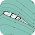# Numerical Methods in Fluid Dynamics

(a-1) Numerical methods - Matlab
Finite Difference Method - Spectral Method

(a-2) Numerical methods - FreeFEM++
Finite Element Method

(a-3) Numerical methods - OpenFOAM
Finite Volume Method

Back to "Learning Materials Directory"

Submitted from Austria

#### 3 comments:

1.Mathematically, the pressure has no boundary conditions, but in the fractional step method, dp/dn=0 is applied to solve the pressure Poisson equation \nabla^2 p=1/dt div u* (here u* is an intermediate velocity). Hence, my question is: do the results by the fractional step method be equivalent to that by the original NS equations?

1.The boundary condition used for the pressure, as you wrote, is not a physical condition. It was introduced, following Chorin, "Numerical Solution of the Navier-Stokes Equations" (1968), as a condition that could reproduce the previous simulations done with the artificial compressibility method. Numerical tests show that such an additional condition gives very robust results in good agreement with experiments and it is largely employed. For high-order methods, a correction of this condition is adopted.

2.This comment has been removed by the author.